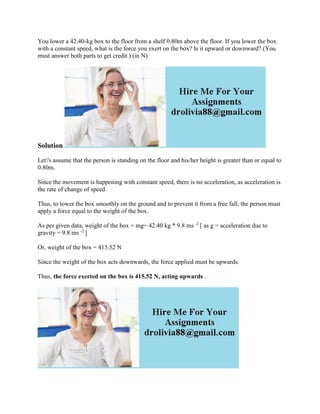Ce diaporama a bien été signalé.
Le téléchargement de votre SlideShare est en cours. ×

# You lower a 42-40-kg box to the floor from a shelf 0-80m above the flo.docx

Publicité
Publicité
Publicité
Publicité
Publicité
Publicité
Publicité
Publicité
Publicité
Publicité
PublicitéChargement dans…3
×

## Consultez-les par la suite

1 sur 1 Publicité

# You lower a 42-40-kg box to the floor from a shelf 0-80m above the flo.docx

You lower a 42.40-kg box to the floor from a shelf 0.80m above the floor. If you lower the box with a constant speed, what is the force you exert on the box? Is it upward or downward? (You must answer both parts to get credit.) (in N)
Solution
Let\'s assume that the person is standing on the floor and his/her height is greater than or equal to 0.80m.
Since the movement is happening with constant speed, there is no acceleration, as acceleration is the rate of change of speed.
Thus, to lower the box smoothly on the ground and to prevent it from a free fall, the person must apply a force equal to the weight of the box.
As per given data, weight of the box = mg= 42.40 kg * 9.8 ms -2 [ as g = acceleration due to gravity = 9.8 ms -2 ]
Or, weight of the box = 415.52 N
Since the weight of the box acts downwards, the force applied must be upwards.
Thus, the force exerted on the box is 415.52 N, acting upwards .
.

You lower a 42.40-kg box to the floor from a shelf 0.80m above the floor. If you lower the box with a constant speed, what is the force you exert on the box? Is it upward or downward? (You must answer both parts to get credit.) (in N)
Solution
Let\'s assume that the person is standing on the floor and his/her height is greater than or equal to 0.80m.
Since the movement is happening with constant speed, there is no acceleration, as acceleration is the rate of change of speed.
Thus, to lower the box smoothly on the ground and to prevent it from a free fall, the person must apply a force equal to the weight of the box.
As per given data, weight of the box = mg= 42.40 kg * 9.8 ms -2 [ as g = acceleration due to gravity = 9.8 ms -2 ]
Or, weight of the box = 415.52 N
Since the weight of the box acts downwards, the force applied must be upwards.
Thus, the force exerted on the box is 415.52 N, acting upwards .
.

Publicité
Publicité

### You lower a 42-40-kg box to the floor from a shelf 0-80m above the flo.docx

1. 1. You lower a 42.40-kg box to the floor from a shelf 0.80m above the floor. If you lower the box with a constant speed, what is the force you exert on the box? Is it upward or downward? (You must answer both parts to get credit.) (in N) Solution Let's assume that the person is standing on the floor and his/her height is greater than or equal to 0.80m. Since the movement is happening with constant speed, there is no acceleration, as acceleration is the rate of change of speed. Thus, to lower the box smoothly on the ground and to prevent it from a free fall, the person must apply a force equal to the weight of the box. As per given data, weight of the box = mg= 42.40 kg * 9.8 ms -2 [ as g = acceleration due to gravity = 9.8 ms -2 ] Or, weight of the box = 415.52 N Since the weight of the box acts downwards, the force applied must be upwards. Thus, the force exerted on the box is 415.52 N, acting upwards .